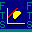Restart to return homeFree Cash Flow Firm Valuation Module

Welcome to the Firm Valuation Module.   This module lets you compute the intrinsic value of a stock using a two stage growth model applied to free cash flows estimated from the latest financial statements available on the web. The module will automatically retrieve the information from an Excel spreadsheet.  You can strategically link the Excel spreadsheet to sheets that can be downloaded from the Web.

IBM Lesson:

For a comprehensive lesson on how to apply the power contained in the FTS Free Cash Flow Module to a real world valuation problem click on: IBM Lesson now.

Aside: For the IBM Lesson you may want to first save the Excel template by clicking on the template, open in Excel (preferably not the Browser) fcfeIBMExample.xls as the workbook and "IBM Example" is the spreadsheet in this workbook that contains the spreadsheet that the IBM lesson refers to.

This lesson also contains a data template for working with the module.

Caution:  This intrinsic value number using the module's default settings should be viewed as a first pass estimate of the intrinsic value.  A second strength of the module is that it permits the user to override any of the default inputs and assumptions with inputs from your own additional research.  This permits a lot of flexibility for conducting "what if" analysis.

What is the two stage growth model using free cash flows?

This model calculates the intrinsic value of a stock as the present value of future free cash flows to equity assuming two growth rates.  In stage I free cash flow to equity is assumed to grow at an abnormal (either high or low) rate for some finite number of years (n where the default value of n is 10).  In stage II free cash flow to equity is assumed to grow at some normal rate from year n+1 in perpetuity.

The concept of free cash flow is cash that could be paid as a dividend without affecting the value of existing operations.  That is, the constraints are cash that is available to shareholders after preserving the firm's investment and financing decisions.  In this sense it can be "freely distributed."

Two major advantages of this concept are:

i.  the close relationship between free cash flows and the concept of economic income, and

ii. the wider applicability of the free cash flow approach when compared to a traditional dividend growth model of intrinsic value.

For example, a traditional dividend growth model cannot be applied to many technology stocks that pay zero dividends, whereas free cash flows can still be estimated and used to value such firms.

How Do I Get Started?

First, you use the module to a) retrieve data from web, b) calculate free cash flows and intrinsic value using this data, and finally c) examine and modify where necessary major input assumptions.

Objective a) is achieved by following three simple steps:

Step 1:  First set up your spreadsheet.  The detailed IBM lesson above provides complete details.

Step 2:  The Excel Spreadsheet described in this lesson provides the set of numbers required as inputs into the calculator plus the lesson describes how to estimate these numbers from the financial statements and other information available from the web.

OS Financial Trading System, PO Box 11356, Pittsburgh, PA 15238 USA, Phone 1-800-967-9897, Fax 1-412-967-5958, Email fts@ftsweb.com,